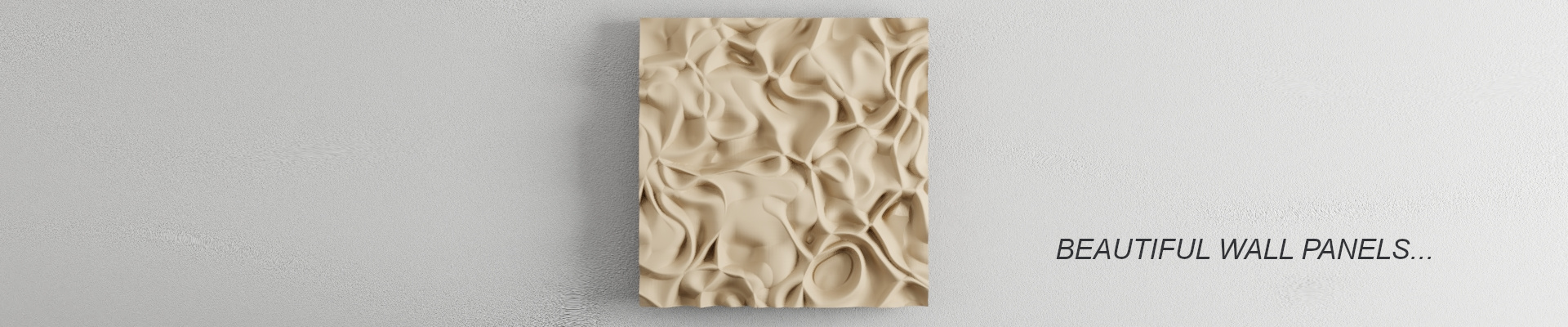Controlled circle packing: make it real

How to use the differential line algorithm to create a decorative tile with CNCI have finally found a simple way to use the circle packing algorithm to “make something real”: a nice wood tile.

In order to achieve this result, similarly to how we did with Differential line growth: make it real, I added few export functions in our `Pack` class:

```String getSaveName() {
return  day()+""+hour()+""+minute()+""+second();
}

void exportDXF() {
String exportName = getSaveName()+".dxf";
PGraphics pg = createGraphics(width, height, DXF, exportName);
pg.beginDraw();
for (int i=0; i<circles.size(); i++) {
Circle p = circles.get(i);
}
pg.endDraw();
pg.dispose();
pg.endRaw();

println(exportName + " saved.");
}

void dxfCircle(float x, float y, float r, float detail, PGraphics pg) {
float inc = TWO_PI / detail;
float px = x +cos(0)*r/2;
float py = y +sin(0)*r/2;
for (float a=inc; a<TWO_PI; a+=inc) {
float x1 = x +cos(a)*r/2;
float y1 = y +sin(a)*r/2;
pg.line(px, py, x1, y1);
px=x1;
py=y1;
}
}

void exportSVG() {
String exportName = getSaveName()+".svg";
PGraphics pg = createGraphics(width, height, SVG, exportName);
pg.beginDraw();
for (int i=0; i<circles.size(); i++) {
Circle p = circles.get(i);
}
pg.endDraw();
pg.dispose();
println(exportName + " saved.");
}

void displayCircle(int i) {
circles.get(i).display();
}```

In this way, by calling an export method (for example via `keyPressed()`) I can get a usable dxf of svg file. With that I went to Voxelizer and select the CNC milling workflow for my ZMorph 2SX, set up the g-code and less than an hour later I had this nice-looking tile 😉